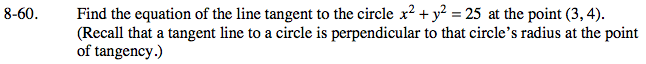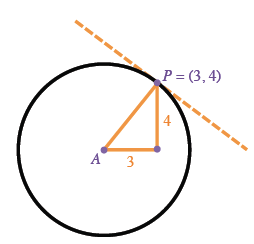### Home > PC > Chapter 8 > Lesson 8.1.4 > Problem8-60

8-60.Draw a diagram of the situation.

Find the slope of the radius, AP.

Find the slope of the tangent line.

It is the negative reciprocal of the slope of the radius found above.

Now you have the point of tangency and the slope. Write the equation using the point-slope form.$\frac{\text{rise}}{\text{run}}=\frac{4}{3}$

$(y-4)=-\frac{3}{4}(x-3)$